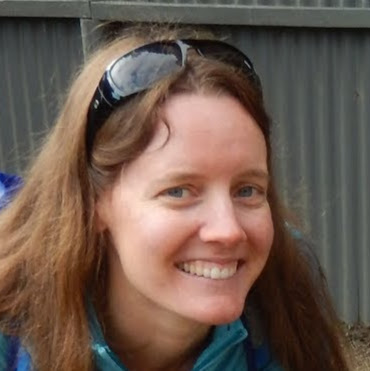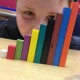# Spatial Puzzles: Cuisenaire Cover-ups

Foundational to almost every aspect of mathematics is the idea that things can be broken down into pieces or units in a variety of ways, and then be recomposed again. For example, the number 10 can be thought of as 2 groups of 5, or 5 groups of 2, or a 7 and a 3, or two-and-one-half and seven-and-one-half…

Earlier this year I shared a post discussing how we might decompose and recompose numbers to do an operations (subtraction). But, I would like us to consider why some students are more comfortable decomposing and recomposing, and how we might be aiming to help our students early with experiences that might promote the kinds of thinking needed.

Doug Clements and Julie Sarama have looked at the relationship between students’ work with space and shapes with students understanding of numbers.

“The ability to describe, use, and visualize the effects of putting together and taking apart shapes is important because the creating, composing, and decomposing units and higher-order units are fundamental mathematics. Further, there is transfer: Composition of shapes supports children’s ability to compose and decompose numbers”

Contemporary Perspectives on Mathematics in Early Childhood Education p.82, Clements and Sarama

The connection between composing and decomposing shapes and numbers is quite exciting to me. However, I am also very interested in the meeting place between Spatial tasks (composing/decomposing shapes) and Number tasks that involve composing and decomposing.

A few years ago I found a neat little puzzle in a resource called The Super Source called “Cover the Giraffe”. The idea was to cover an image of a giraffe outline using exactly 1 of each size of cuisenaire rods. The task, simple enough, was actually quite difficult for students (and even for us as adults). After using the puzzle with a few different classes, I decided to make a few of my own.

After watching a few classrooms of students complete these puzzles, I noticed an interesting intersection between spatial reasoning, and algebraic reasoning happening…. First, let me share the puzzles with you:

## Objective:

To complete a Cuisenaire Cover-Up puzzle, you need exactly 1 of each colour cuisenaire rod. Use each colour rod once each to completely fill in the image.

Below are the 5 puzzles:

## Assessment Opportunities

Knowing what to look for, helps us know how to interact with our students.

• Which block are students placing first? The largest blocks or the smallest?
• Which students are using spatial cues (placing rods to see which fits) and which students are using numerical cues (counting units on the grid)? How might we help students who are only using one of these cueing systems without over-scaffolding or showing how WE would complete the puzzle?
• How do our students react when confronted with a challenging puzzle?
• Who is able to swap out 1 rod for 2 rods of equivalent length (1 orange rod is the same length as a brown and red rod together)?
• Which of the following strands of proficiency might you be noting as you observe students:

## Questions to Reflect on:

• Why might you use a task like this? What would be your goal?
• How will you interact with students who struggle to get started, or struggle to move passed a specific hurdle?
• How might these puzzles relate to algebraic reasoning? (try to complete one with this question in mind)
• How are you making the connections between spatial reasoning and algebraic reasoning clear for your students to see? How can these puzzles help?
• How might puzzles allow different students to be successful in your class?

If interested in these puzzles, you might be interested in trying:

## 5 thoughts on “Spatial Puzzles: Cuisenaire Cover-ups”

1.Kelsey Clinton says:

How would you use algebra in this exactly? I don’t have cuisenaire rods with me right now to try it out but I can’t quite figure out how to connect this with algebra

Like

1.Mark Chubb says:

Algebraic reasoning involves much more than “solving for x”. At the heart of algebraic reasoning lies the idea of generalizing (including finding patterns related to calculations).

These problems are interesting only because you are allowed to use one of each colour rod. Here students will come to see a row of 10 and assume that the ways to fill the row is to use one orange (length of 10) or one white (1) and one blue (9) or one red (2) and one brown (8)… and be able to generalize about the equivalencies related to 10. Other parts of the puzzle will have other varying lengths that will require similar patterns to be considered.
Solving the problems might require informal algebraic reasoning, but symbolic representations here can/should be discussed as students are maturing and making sense of the task. Ideally, if you used these problems, you would consider how/what you would want to consolidate so your students would be able to discuss the mathematics involved in the problem (not just solve it).
Hopefully students will be able to note whether they thought about the largest spaces or smallest spaces first, and consider which possibilities they tried first ( 10+2=9+3=8+4=7+5 ).
Does this make sense?

Like